StatLect

Estimate

The term "estimate", or "statistical estimate" is used in the theory of statistical estimation. It is assumed that some data observed by the statistician have been generated by an unknown probability distribution. The statistician uses the data to infer some quantifiable characteristics of this probability distribution (by quantifiable we mean that they can be described by numbers). An estimate of the value of one of these characteristics is the statistician's best guess of it.

The theory of statistical estimation is concerned with devising methods to produce estimates (called estimation methods), and with defining coherent criteria to compare these methods and to judge their appropriateness (called decision criteria).Parametric estimate

A common conceptual framework for statistical estimation comprises the following elements:

• a vector of observed data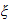;

• a set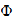of probability distributions that could have generated the data;

• a correspondence that associates the distributions into vectors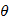, called parameters, that belong to a parameter space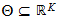;

• a method, called estimation method, that uses the observed data to produce an estimate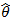of the true parameter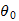(the parameter associated to the distribution that actually generated the data).

Such a framework is called parametric framework because it reduces the problem of identifying the data-generating probability distribution to the problem of identifying a parameter (a vector of numbers).

Estimate vs estimator

An estimation method can be seen as a rule that associates an estimate to each vector of data that can possibly be observed. Because the data is random, this implies that the estimate is a random vector from an ex-ante perspective. Under this perspective, such a random vector is called estimator, in order to distinguish it from its realization, that is, the estimate associated to the data that has actually been observed.

Point vs interval estimate

In so-called point estimation, the estimate of the parameter of interest is a single value. However, the statistician might be interested in producing a whole range of parameter values that he deems possible (or likely) after having observed the data. Such a range of values is called an interval estimate (or set estimate).

More details

More details about estimation can be found in the lectures entitled Statistical inference, Point estimation and Set estimation.